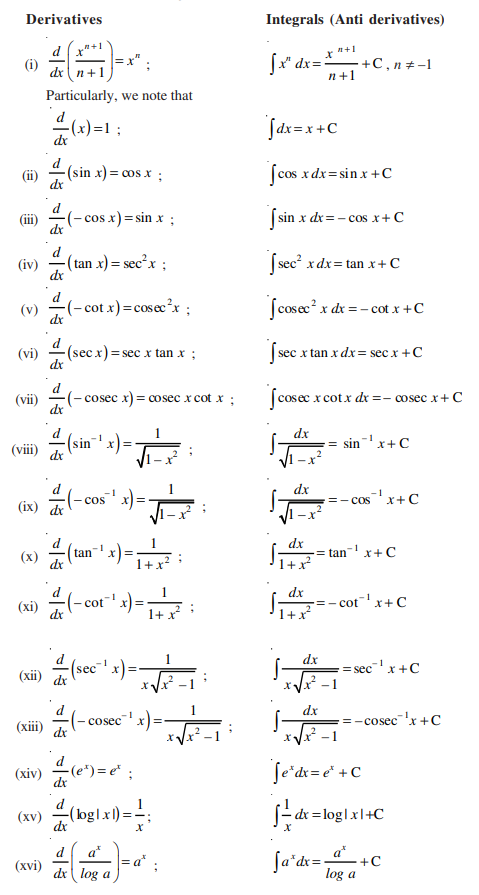Checkout JEE MAINS 2022 Question Paper Analysis : Checkout JEE MAINS 2022 Question Paper Analysis :

# Integrals For Class 12

Integrals class 12, chapter 7 deals with the study of definite and indefinite integrals and their elementary properties. The development of integral calculus arises out to solve the problems of the following types:

• The problem of finding the function whenever the derivatives are given.
• The problem of finding the area bounded by the graph function under certain conditions.

These two problems lead to the development of integral calculus (definite integral and indefinite integral).

## Integrals Class 12 Concepts

The topics and subtopics covered under integrals class 12 Maths are:

• Introduction
• Integration as an inverse process of differentiation
• Geometrical interpretation of indefinite integral
• Some properties of indefinite integrals
• Comparison between differentiation and integration
• Methods of integration
• Integration by substitution
• Integration using trigonometric identities
• Integrals for some particular functions
• Integration by partial fractions
• Integration by parts
• Integral of the type
• Integrals for some more types
• Definite integral
• Definite integral as a limit of a sum
• The fundamental theorem of calculus
• Area function
• The first fundamental theorem of integral calculus
• The second fundamental theorem of integral calculus
• Evaluation 0f definite integrals by substitution
• Some properties of definite integrals

Learn: Integral

## Integrals Class 12 Notes

We are already aware that if a function f(x) is differentiable on an interval I, it’s derivative f’ (x) exists at each point of I. Now the question arises: if the function’s derivative is known to us, is it possible to obtain the function. The answer to this question is yes. Integration ( or antiderivative of a function) makes it possible to obtain the original function.

Integration as an Inverse Process of Differentiation

Integration is called the inverse process of differentiation. Instead of differentiating a function, we are given the function’s derivative and asked to find its primitive, i.e., the original function. Such a process is called integration or anti-differentiation.

### Antiderivative Functions

Let us have a look at various antiderivative of functions.### Integral Types

The integral calculus is of the two forms, namely

(i) Indefinite Integral

(ii) Definite Integral

In an indefinite integral, the range of the function is not defined, thus the value of the function obtain is followed by a constant value ‘c.

Whereas in a definite integral, the range of the function is well defined, thus it gives a well-defined function.

The integration is denoted by

$$\begin{array}{l}(\int)\end{array}$$
.

### Indefinite Integrals

Some of the important concepts of indefinite integrals are as follows:

### Methods of Integration

In calculus, different methods exist to perform the integration, i.e. to find the integral of a function. These methods are used to find the antiderivatives of complex functions. They are:

Integrals of Particular Functions

Some of the important formulas to find the integrals of particular functions are given below:

Also, check: Integral of particular function

### Definite Integral

A definite integral is denoted by ∫ab f(x) dx, where a is called the lower limit of the integral and b is called the upper limit of the integral.

### Fundamental Theorem of Calculus

Fundamental theorem of calculus contains two important theorems namely:

First fundamental theorem of integral calculus

Let f be a continuous function on the closed interval [a, b] and let A (x) be the area function. Then A′(x) = f (x), for all x ∈ [a, b].

Second fundamental theorem of integral calculus

Let f be a continuous function defined on the closed interval [a, b] and F be an antiderivative of f. Then ∫ab f(x) dx= [F(x)]ab = F(b) – F(a)